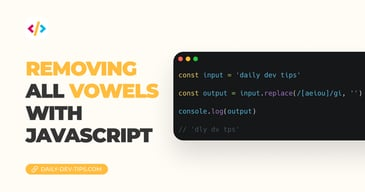Subscribe

# Vanilla JavaScript String to Number

✍️

Three different ways to convert a String into a number

4 Aug, 2020 · 2 min read

Sometimes we want to convert a number to a string; in my latest code piece, a limit was set on a data attribute. This comes true as a string, so how do we convert this to a number in `JavaScript`?

In this case, there are not one but three valid and supported methods of converting our string to a number.

• Number
• parseInt
• parseFloat

Number is a generic way of converting. It's the stricter check. Here are some examples with outputs:

``````<!-- Number -->
console.log(Number(`1337`)); // 1337
console.log(Number(`13.37`)); // 13.37
console.log(Number(`13leet37`)); // NaN
console.log(Number(`13,37`)); // NaN``````

As you can see, it works on actual numbers, but as soon as we use other characters or a comma, it won't work.

ParseInt converts our string into an integer non-rounded. It has two arguments, the input and the `radix`, which is the base number. For us, the default is 10

``````<!-- ParseInt -->
const text1 = '1337';
const text2 = '13.37';
const text3 = '13leet37';
const text4 = '13,37';
console.log(parseInt(text1, 10)); // 1337
console.log(parseInt(text2, 10)); // 13
console.log(parseInt(text3, 10)); // 13
console.log(parseInt(text4, 10)); // 13``````

The downside can be it's rounding everything, so let's look at ParseFloat.

As mentioned, the parseFloat is used to return stuff with decimals.

``````<!-- ParseFloat -->
const text1 = '1337';
const text2 = '13.37';
const text3 = '13.3leet37';
const text4 = '13,37';
console.log(parseFloat(text1, 10)); // 13.37
console.log(parseFloat(text2, 10)); // 13
console.log(parseFloat(text3, 10)); // 13.3
console.log(parseFloat(text4, 10)); // 13``````

Very cool method!

See all these in action on Codepen.

See the Pen Vanilla JavaScript String to Number by Chris Bongers (@rebelchris) on CodePen.

Tweet this tip

### Removing all vowels with JavaScript

3 Dec, 2022 · 2 min read### 10 games to learn JavaScript

2 Dec, 2022 · 3 min readJoin 2101 devs and subscribe to my newsletter

• 1000 articles written
• 2101 devs subscribed
• 529116 words written